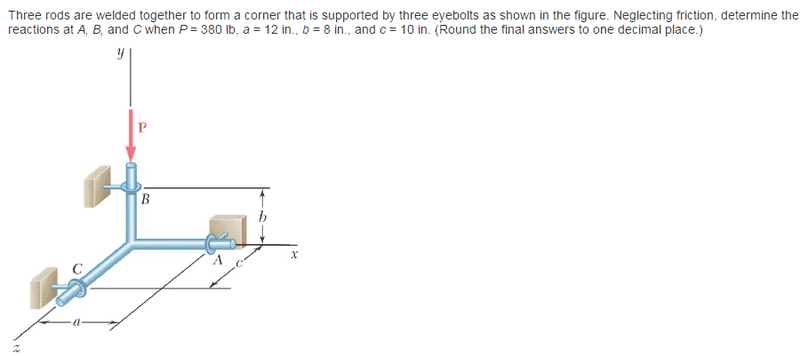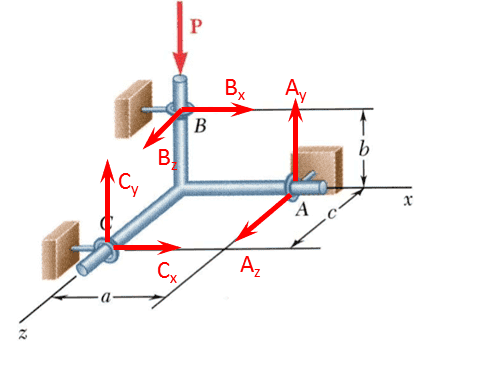# Statics in 3d question

## Homework Statement## The Attempt at a Solution

Free body diagramSetting moment at the origin = 0

(Rb X B) + (Ra X A) + (Rc X C) = 0

solving the 3 determinants and setting the coefficients of i, j, and k to 0, I get:

8Bz - 10 Cy = 0

-12 Az + 10 Cx = 0

12 Ay - 8Bx = 0

I have too many unknowns.

summing forces :

∑Fx = Bx + Cx = 0

∑Fy = Ay + Cy - P = 0 => Ay + Cy = 380

∑Fz = Az + Bz = 0

I still have too many unknowns.

Any suggestions?

SteamKing
Staff Emeritus
Homework Helper

## Homework Statement

View attachment 99005

## The Attempt at a Solution

Free body diagram
View attachment 99006

Setting moment at the origin = 0

(Rb X B) + (Ra X A) + (Rc X C) = 0

solving the 3 determinants and setting the coefficients of i, j, and k to 0, I get:

8Bz - 10 Cy = 0

-12 Az + 10 Cx = 0

12 Ay - 8Bx = 0

I have too many unknowns.

summing forces :

∑Fx = Bx + Cx = 0

∑Fy = Ay + Cy - P = 0 => Ay + Cy = 380

∑Fz = Az + Bz = 0

I still have too many unknowns.

Any suggestions?
Just like you would do when solving a 2-D problem, it is better to write a moment equation using one of the three rings as the reference, rather than picking another arbitrary point like the origin. Using one of the rings as the reference for the moment equation eliminates one of the forces, and you should be able to solve for the remaining forces using the two equations of statics which you are allowed to write.

Just like you would do when solving a 2-D problem, it is better to write a moment equation using one of the three rings as the reference, rather than picking another arbitrary point like the origin. Using one of the rings as the reference for the moment equation eliminates one of the forces, and you should be able to solve for the remaining forces using the two equations of statics which you are allowed to write.
you mean for example, taking ∑Mc = 0 along the x-axis : (Bz)(8) + (Ay)(15.62) - (P)(10in) = 0

(Bz)(8) + (Ay)(15.62) = (380)(10) = 3800

Last edited:
actually, I was wrong, I had all I needed already, it was just A LOT of substituting but in the end, I got Ay = Cy
plugging into Ay + Cy = 380

Ay = Cy = 190

A lot of subbing to find Ay = Cy

12 Ay = -8 Cx = -8(12/10 Az) = 8(12/10) Bz = . . . and so on